Nishant Malhotra Founder of Middle Road OPC Pvt Ltd & The middle Road platform talks about the startup

### Ahoy Ahoy-Ahoy!

Welcome to the Kickass platform enabling social change & impact

# Term Structure of Interest Rates

Term Structure of Interest Rates is a sample of the publications under Tutorials and part of the ongoing tutorial section under Valuation with a focus on Fixed Income securities.

Term structure of interest rates remains one of the most important tools in the bond market for pricing fixed income securities.  This educational note is a primer to have a better understanding on this subject.

### The series on Term Structure of Interest Rates discusses the following topics

• Bonds Simplified : A brief overview of bonds which a look at yield, YTM (Yield to Maturity), yield curve and various types of yield curve
• Understanding price and yield relationship
• Overview and valuation of zero coupon bonds and deriving theoretical spot rate curve from the par yield curve
• Calculating price of the bonds using spot rates and YTM
• Understanding forward rates, calculating forward rates and much more

In the parlance of bond valuation spot rates have the utmost role as determinants of building blocks of understanding the term structure of interest rates. The price of the bond is the present value of the cash flows. The cash flows of bonds can be considered zero-coupon bonds. Zero-coupon bonds are not coupon securities but pay interest in the form of discounting the issue price to the face value. The yields of these zero-coupon bonds are also known as spot rates. Spot rates are discount rates of a Single Future Cash Flow like a zero-coupon bond.1

To derive the spot rate of each treasury security of a particular time it is important to known the spot rate of each of these securities. Before we understand how to derive a theoretical spot rate curve, we delve into a few basics.A bond has a face value or par value and coupon paying securities are issued at the face value and begin trading. A bond has a term to maturity during which term the issuer (the originator of the bond) promises to fulfill the conditions of the obligations attached to the bond. These include paying a coupon rate i.e. interest on the face value of the bond to the bondholder. Government treasuries like the US pay semiannual rates of interest although types of interest payments vary. According to classification by Fabozzi bonds with maturity between 1 to 5 years are short-term securities although for the tutorial’s bonds with maturities 3 to 6 months will also be referred to as short term securities while medium term ranges from 5 to 12 years. Long term bonds are of duration 20 or 30 years but usually on the run US treasury securities i.e. treasury securities issued recently range from 2,5 and 10 in intermediate term to 30 years in the long term. In the fig, yield refers to the current yield of the bond.

On the run securities and selected off the run securities or/are used to derive a theoretical spot rate curve from par yield curve of securities through Bootstrapping. This technique will be explained today.

To know more about types of issuers, selected various kinds of bonds and much more refer to the tutorial on Global Bond. The educational module does not include bonds with embedded options which will be discussed at a later date.

Bonds are often quoted in term of its current yield and yield to maturity.  As bonds trade upon issuance, the yields of the bond change.  The yield of the bonds is inversely related to price of the bonds. The price of the bonds is the present value of all the cash flows discounted at either the spot rates of respective time periods or yield to maturity.

###### Left Pic: Middle Road OPC Pvt Ltd

To have a better understanding of present value valuation refer to the module on  Concept of Present and Future Value.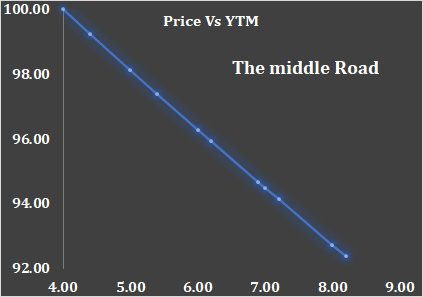A plain vanilla bond cash flows are divided into interest coupon and maturity value.Consider government security paying a semi-annual coupon interest, the value of the bond can be derived by calculating the present value of the bond by discounting the cash flows using the appropriate discount rate. The discount rate can be the YTM or spot rates from a theoretical spot rate curve. Left graph: Price YTM graph plot for a 2 year 4% (annualized) semi-annual payment government security. For understanding, a detailed valuation of the bonds refers to the excel sheet attached in the tutorial read here.

The cash flows for 5 periods are calculated using the coupon rate for a bond with par 100 i.e. 2 here.

The cash flows are discounted using YTM as the discount rate for calculating the prices at different YTMs. The price of the bond is clearly inversely related to the yield to maturity.

Note: The higher the discount rate, the lower the present value of the cash flow.

###An important concept to understand here is how par, discount and premium bonds are related to current yield and yield to maturity.  Consider the above example, the coupon rate is 4%. At YTM of 5%, the bond is priced at 98.12. the current yield is 4.08%. Refer to cal. on the left side.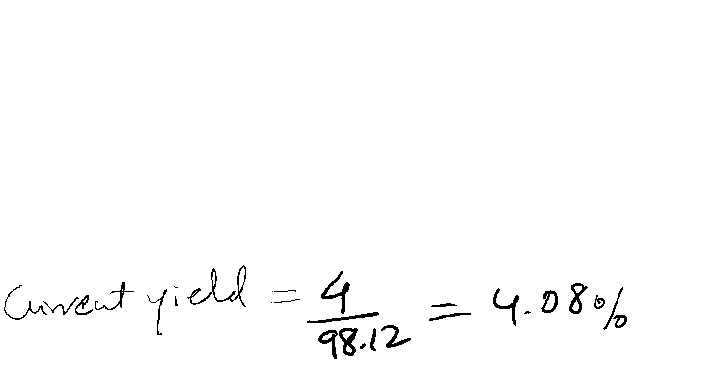At 98.12, the bond is trading at a discount.

For a discounted bond, coupon rate < current yield  < yield to maturity

Similarly,  at 3% YTM, the bond trades at a price of 101.927.

At this price, the current yield is 4/101.927 = 3.92%. When the bond trades at a premium, the coupon rate>current yield> YTM

### # Understanding Yield to Maturity

Single interest rate that discounts all the cash flows from the bond and equates it to the current market price of the bond. YTM is the internal rate of return when the bond is held until maturity.  To calculate YTM there are a couple of assumptions.

First, the bond is held until maturity. Second, the coupons are reinvested at the computed YTM.  The reinvestment of coupons at YTM is a bit stretched since this would mean that the yield curve is flat which is not a usual scenario. The calculation of YTM for a semi annual coupon bond is below. In calculations here the final value is always taken as coupon + maturity value.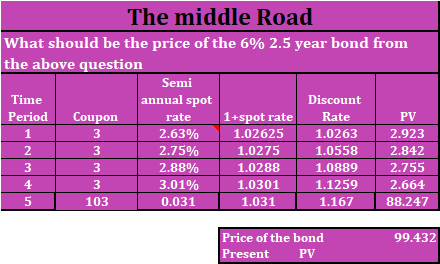In the above example, the cash flows are discounted at YTM. However, it is unreasonable to assume a constant discount rate prevailing at different tome periods.  A better way of understand in the present value of the bond is to use spot rates at different time periods for bond valuation. Consider a semi annual 6%, 2.5 year government security. The par vale of the bond is 100.  Refer to the table below and calculate the price of the bond.  The annual coupon is 6 paid semi annual as 3. Each period is discounted using the respective spot rates.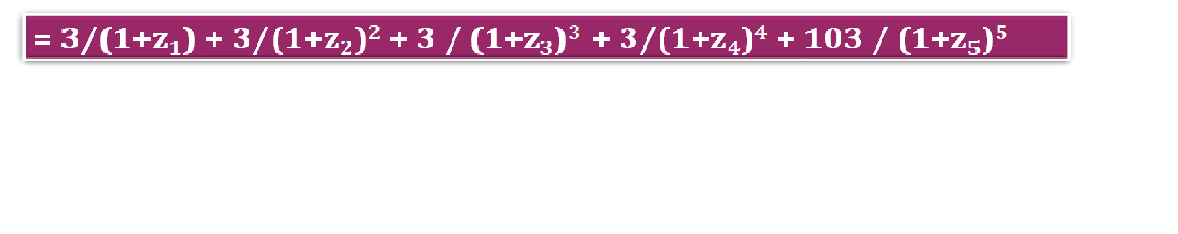Zero Coupon bonds don’t pay any coupons but the yield is calculated as the difference between the issue and maturity price.  In a zero coupon bond, the periods for calculation are doubled.

The price of the bond varies according to coupon, maturity, prevailing market rates and comparable bonds in the market which are of similar credit profile. All government securities are risk free and spot rates of different maturities are used to construct a theoretical spot rate curve which forms the term structure of interest rates.

### # Term Structure of Interest Rates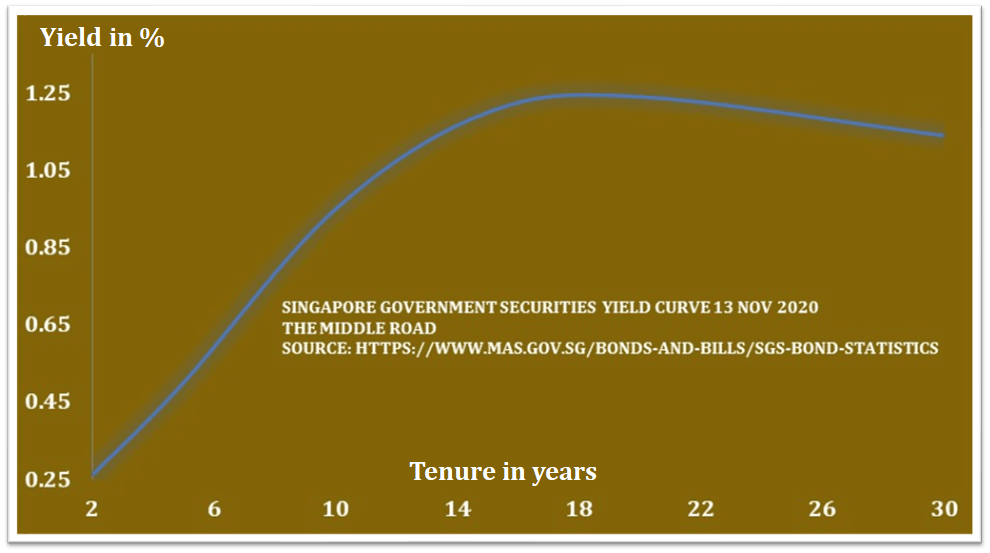###### Above: Normal yield curve Singapore Government Securities Yield Curve | Pic Middle Road OPC Pvt Ltd

The relationship between yield and maturities of comparable bonds of similar credit quality is called the term structure of interest rates and yield curve its graphical description. The yield curve is plotted with risk-free government zero coupon term structure i.e. spot rates/zero-coupon rates of different time durations. The yield curve can also be drawn for government agencies etc. Term structure equates term to maturity to maturities of different bonds of different time horizons with comparable risk profiles. Treasuries are rated risk-free so treasury securities of varying durations serve as a barometer and predictor of economic conditions prevailing with the global economy. The yield curve represents the market remuneration of interest rates concerning the time to maturity of bonds. The yield curve depicts the premium the market is willing to pay for bonds of various maturities keeping other factors constant.

There are four types of yield curve as depicted below.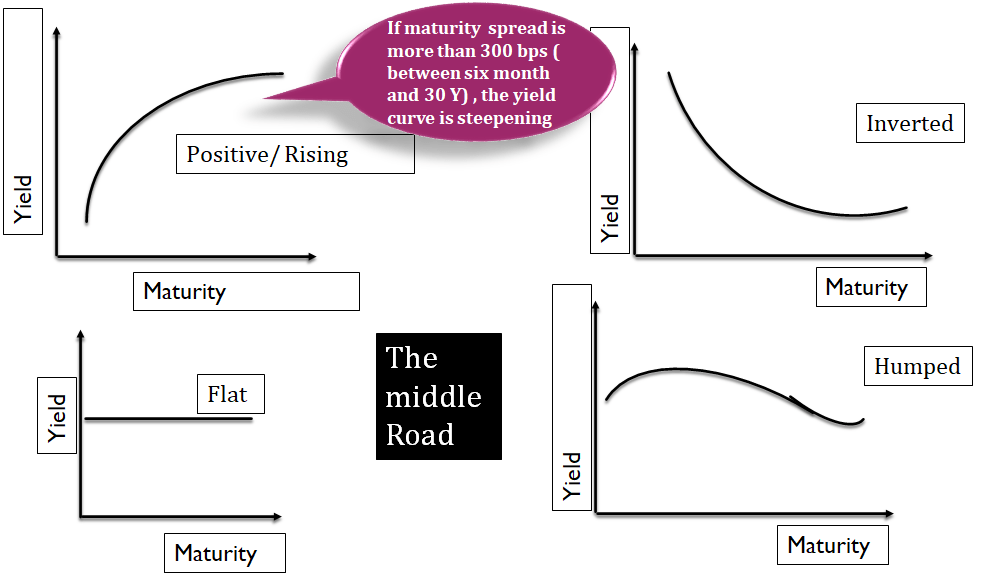###### Pic: Middle Road OPC Pvt Ltd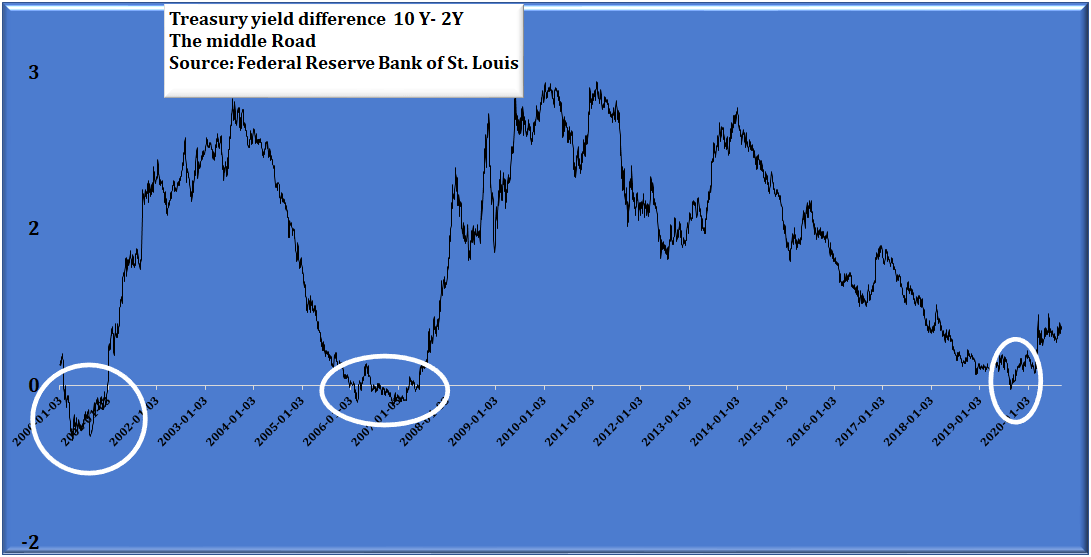Positive or rising yield curve is the most observed with the difference between short term and long term yields less than 300bps based on the definition provided by Fabozzi. Yield curves with differences greater than 300bps are known as steepners. Bonds of longer duration are expected to have a higher coupon rate due to higher volatility and risk adding to a higher risk premium.

Inverted yield curves are usually but a significant predictor of recession ahead. Pic left circled portions of US yield inversions diff 10Y-2Y. Graph: Middle Road OPC Pvt Ltd.

*Post world war 2 inversions predicted seven out of 9 recession Sung Won Sohn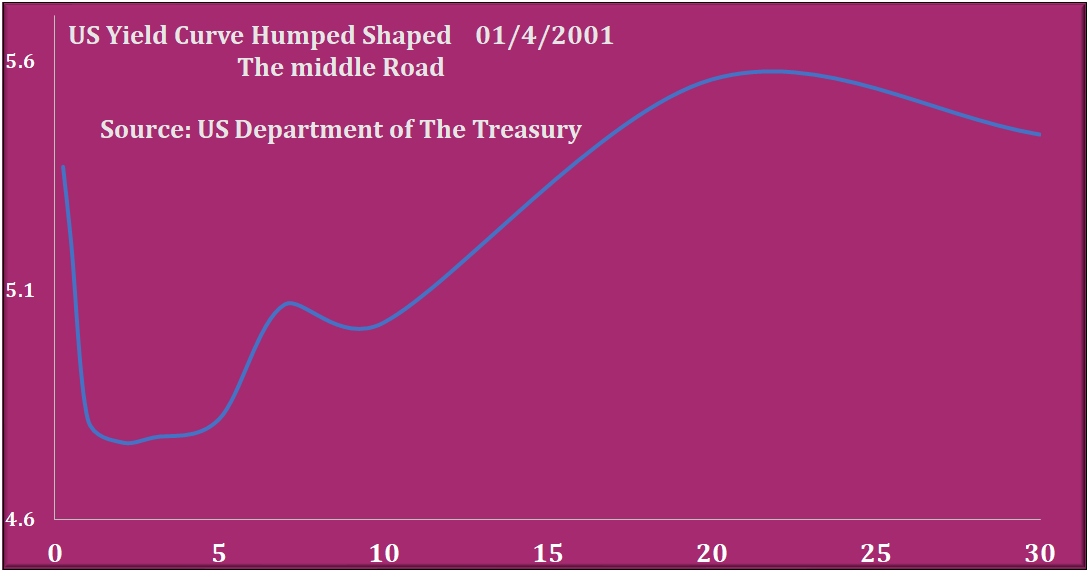Data from Credit Suisse going back to 1978 shows: Source CNBC

• The last five 2-10 inversions have ultimately led to recessions.
• A recession occurs, on average, 22 months following a 2-10 inversion.
• The S&P 500 is up, on average, 12% one year after a 2-10 inversion.
• It’s not until about 18 months after an inversion when the stock market usually turns and posts negative returns.
###### Graph left: Middle Road OPC Pvt Ltd

Based on the information shared above, yield inversions are an excellent indicator for forecasting recession in the US. Similarly, flat and humped shaped yield curves are less frequent but have been observed in the US. Left humped shaped yield curve. In this type of shape bonds of medium maturity have yields less than bonds at the shorter term of the curve. The yields gradually rise for long term bonds before tapering down.

### # Bootstrapping: Calculation of theoretical spot rate curve from a par yield curve

There are multiple ways in which a theoretical spot rate curve can be derived from the par yield curve of Treasury securities. Some of them involve advanced statistical analysis while stripping coupon securities into zero-coupon bonds is not the best way to derive yield due to tax distortions. The most popular way is to use on the run treasury securities along with selected of the run treasury securities called Bootstrapping. US treasury frequently-issued treasuries are Treasury Notes: 2, 5 & 10 and 30-year long term bonds. This leaves a lot of gaps that need to be filled through off-the run treasuries. One simple method is to use the linear interpolation method between two securities of different maturities. However, this method oversimplifies yields of  securities which lie between two maturities.

Bootstrapping is the method used to construct a theoretical yield curve from a par yield curve of treasury securities. US Treasuries only issue zero coupon bonds for six month and 1 year securities, so yields on them are spot rates. Refer to the table on the left. If 6 month and one year spot rates are given, you can construct the spot rate curve for bonds of all term maturity provided you have the par yield curve for the same duration.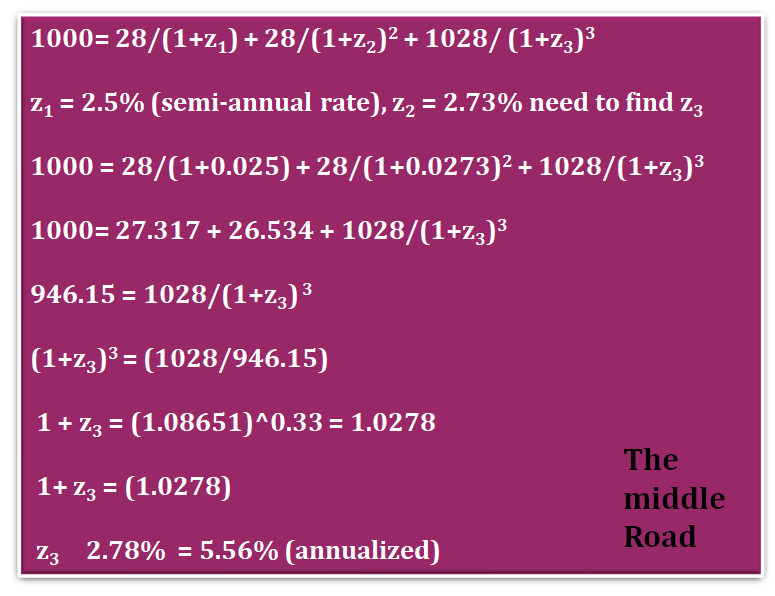At par coupon rate = yield to maturity which is required for deriving spot rates for all  durations. In the present example,  calculate spot rate for 1.5 and 2 year time duration. Calculate spot rate for time period 3 i.e. a theoretical 1.5 year zero coupon bond from the given yield to maturity table

0.5 and 1 year bonds are Zero coupon bonds so consider their yields as spot rates. Consider all securities to have market price equal to its par. Consider Par / Face Value as 1000. For period 1.5 years, YTM is 5.60% annualized so cash flows period for the three periods are 28, 28 , 28+1000. The face value of the bond is 1000. Refer to the excel sheet for detailed calculations.

### # Forward Rates

A theoretical spot rate curve implies a term structure of forward rates. The theoretical spot rate curve, helps to understand the market expectation of future short term rates called forward rates. There are many hypothesis which explains the term structure of forward rates like pure expectations theory, liquidity theory, market segmentation theory etc.  However, a study done by Antti Ilanen found that forward rates had a limited success in explaining future spot rates.

The three main influences on the yield curve are the following.

1. Market expectation of future rate changes
3. Convexity bias

As of now, we will ignore convexity bias as we will come to this when we analyze bond valuation in depth. To understand the importance of forward rates take an example when an investor wants to invest over one year.  The method of calculating a future interest rate either through spot rate or yield curve is called a forward rate. The global macroeconomic outlook is very dynamic and affected by many factors to decisively predict forward rates at for different maturities but market prices future expectation of interest rates into buckets of varied maturities. Forward rates is the interest rate charged between two periods in future contracted today.

Understanding the importance is vital to have a grasp of the underpinnings of global economic conditions within the investment arena. The investor can invest the proceeds in a treasury of 1-year maturity or invest the capital in six-month security and roll over the investment again in six months. However, it would be prudent to understand how the interest rates would be prevailing in six months. If there is an onset of deep recession and the interest rates are going to go down, the investor is better off investing in 1-year security and locking his return. Investor would be even if the returns equal through both the alternatives.### S2 : Since there are two time periods from the present to year 1, the suffix 2

The forward rate is calculated using the formula below.  Refer to the excel sheet here for a comprehensive calculation of forward rates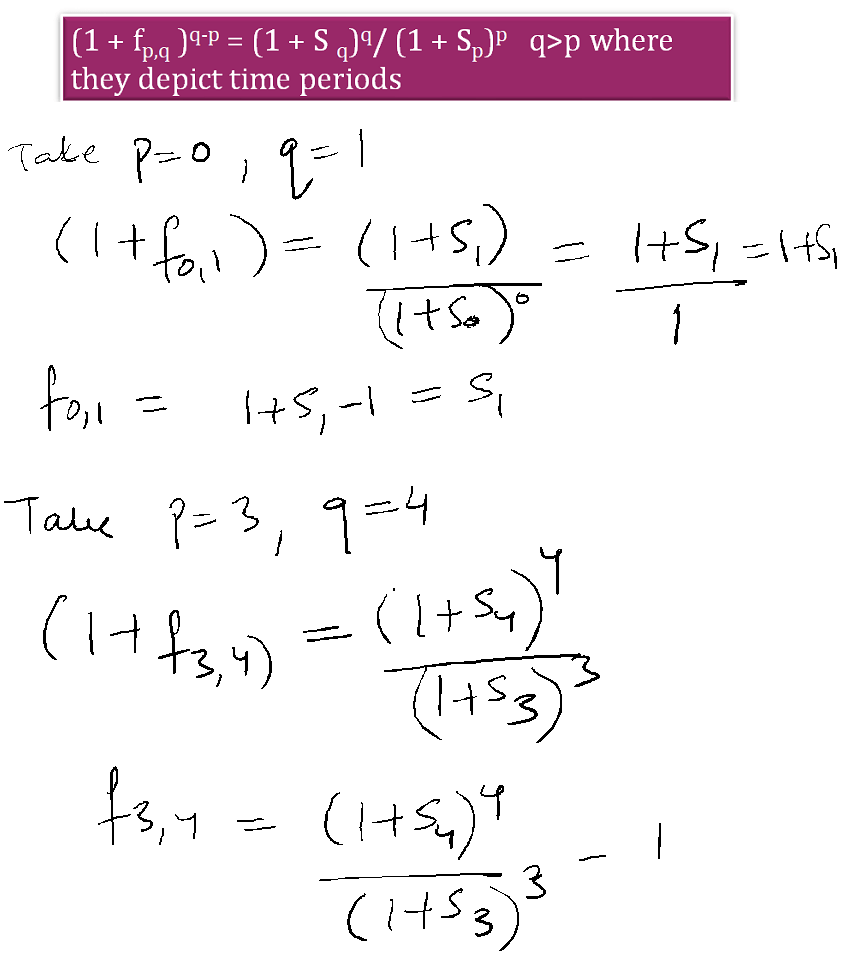### Spot rate the geometric mean of one year forward rates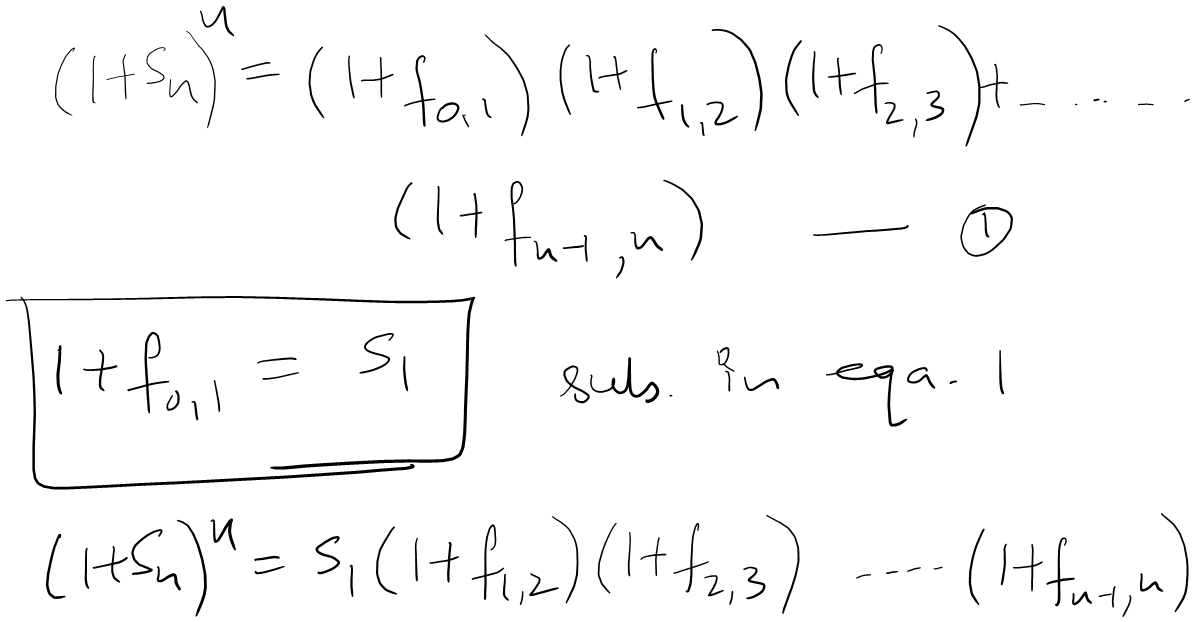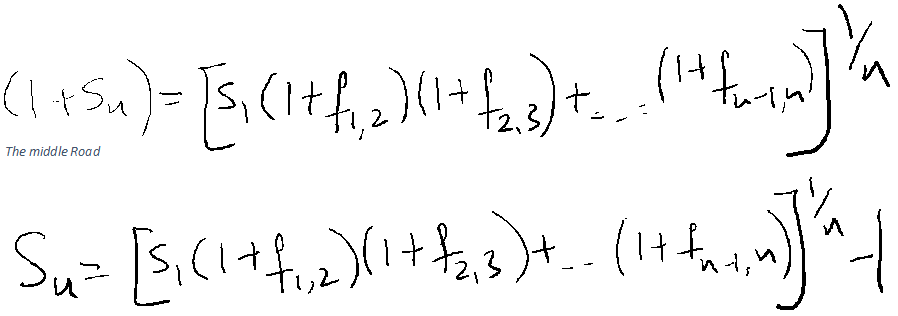Example: An investor wants to invest for two years a sum of 100. If the spot rate S1= 5% (annualized) and the future rate between time period 1 and 2 is f1,2 is 5.90% annualized. What will be the expected return on the capital given the data.

Ans (~111.2).

Question: The one year forward rate are as follows. Find the annualized spot rate for time period 3. ( Ans 5.9%)

 f0,1 5.00% f1,2 5.90% f2,3 6.72%

Note: The excel sheet given is to build on practice problems will be following soon. The sheet also includes implied spot rate one year from now. We will be discussing theories affecting term structure of interest rates, work sheet problems and much more. Convexity concept will be discussed as we go forward. The implied spot rate column should be ignored for now.

For excel sheet refer to the original publication under Tutorial section here.